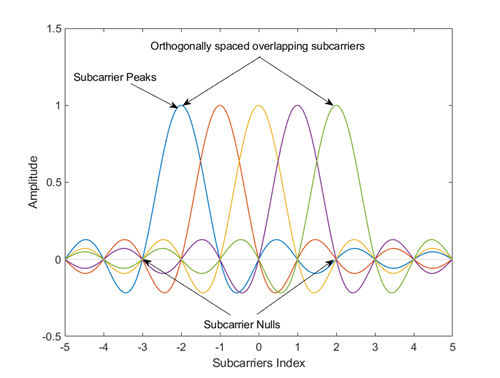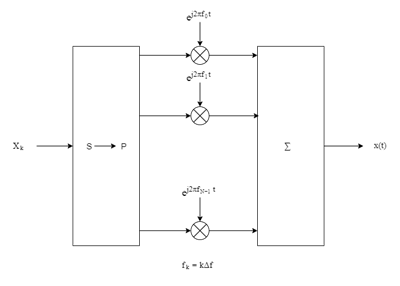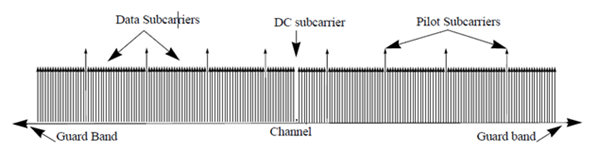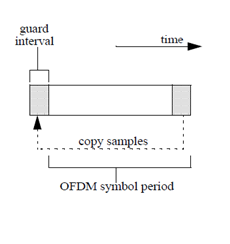# ofdmmod

Modulate using OFDM method

## Syntax

``Y = ofdmmod(X,nfft,cplen)``
``Y = ofdmmod(X,nfft,cplen,nullidx)``
``Y = ofdmmod(X,nfft,cplen,nullidx,pilotidx,pilots)``
``Y = ofdmmod(X,nfft,cplen,___,OversamplingFactor=Value)``

## Description

example

````Y = ofdmmod(X,nfft,cplen)` modulates the frequency-domain input data subcarriers in `X` using the orthogonal frequency division multiplexing (OFDM) method with an FFT size specified by `nfft` and cyclic prefix length specified by `cplen`. For information, see OFDM Modulation.```

example

````Y = ofdmmod(X,nfft,cplen,nullidx)` inserts null subcarriers into the frequency domain input data signal prior to performing OFDM modulation. The null subcarriers are inserted at index locations from 1 to `nfft`, as specified by `nullidx`. For this syntax, the number of rows in the input `X` must be `nfft` – `length(nullidx)`. Use null carriers to account for guard bands and DC subcarriers. For information, see Subcarrier Allocation, Guard Bands and Guard Intervals.```

example

````Y = ofdmmod(X,nfft,cplen,nullidx,pilotidx,pilots)` inserts null and pilot subcarriers into the frequency domain input data symbols prior to performing OFDM modulation. The null subcarriers are inserted at the index locations specified by `nullidx`. The pilot subcarriers, `pilots`, are inserted at the index locations specified by `pilotidx`. For this syntax, the number of rows in the input `X` must be `nfft` – `length(nullidx)` – `length(pilotidx)`. The function assumes pilot subcarrier locations are the same across each OFDM symbol and transmit antenna.```

example

````Y = ofdmmod(X,nfft,cplen,___,OversamplingFactor=Value)` specifies the optional oversampling factor name-value argument in addition to input arguments in previous syntaxes. The oversampling factor for an upsampled output signal must be specified as a positive scalar, and the products (`OversamplingFactor`×`nfft`) and (`OversamplingFactor`×`cplen`) must both result in integers. For example, `ofdmmod(X,nfft,cplen,OversamplingFactor=2)` upsamples the output signal by a factor of two. The default value for `OversamplingFactor` is `1`. TipIf you set the oversampling factor to an irrational number, specify the fractional value. For example, with an FFT length of `12` and an oversampling factor of `4/3`, their product is the integer `16`. However, rounding `4/3` to `1.333` when setting the oversampling factor results in a noninteger product of `15.9960`, which results in a code error. ```

## Examples

collapse all

OFDM-modulate a fully packed input over two transmit antennas.

Initialize input parameters, generate random data, and perform OFDM modulation.

```M = 16; % Modulation order for 16QAM nfft = 128; % Number of data carriers cplen = 16; % Cyclic prefix length nSym = 5; % Number of symbols per RE nt = 2; % Number of transmit antennas dataIn = randi([0 M-1],nfft,nSym,nt); qamSig = qammod(dataIn,M,'UnitAveragePower',true); y1 = ofdmmod(qamSig,nfft,cplen);```

Apply OFDM modulation assigning null subcarriers.

Initialize input parameters and generate random data.

```M = 16; % Modulation order for 16QAM nfft = 64; % FFT length cplen = 16; % Cyclic prefix length nSym = 10; % Number of symbols per RE nullIdx = [1:6 33 64-4:64]'; numDataCarrs = nfft-length(nullIdx); inSym = randi([0 M-1],numDataCarrs,nSym);```

QAM modulate data. Perform OFDM modulation.

```qamSig = qammod(inSym,M,'UnitAveragePower',true); outSig = ofdmmod(qamSig,nfft,cplen,nullIdx);```

Perform OFDM modulation to input frequency domain data signal varying cyclic prefix length applied to each symbol.

Initialize input parameters and generate random data.

```M = 16; % Modulation order for 16QAM nfft = 64; cplen = [4 8 10 7 2 2 4 11 16 3]; nSym = 10; nullIdx = [1:6 33 64-4:64]'; numDataCarrs = nfft-length(nullIdx); inSym = randi([0 M-1],numDataCarrs,nSym);```

QAM modulate the data symbols and perform OFDM modulation to the QAM signal.

```qamSig = qammod(inSym,M,UnitAveragePower=true); outSig = ofdmmod(qamSig,nfft,cplen,nullIdx);```

Apply OFDM modulation to a QPSK signal that is spatially multiplexed over two transmit antennas.

Initialize input parameters and generate random data for each antenna.

```M = 4; % Modulation order for QPSK nfft = 64; cplen = 16; nSym = 5; nt = 2; nullIdx = [1:6 33 64-4:64]'; pilotIdx = [12 26 40 54]'; numDataCarrs = nfft-length(nullIdx)-length(pilotIdx); pilots = repmat(pskmod((0:M-1).',M),1,nSym,2); ant1 = randi([0 M-1],numDataCarrs,nSym); ant2 = randi([0 M-1],numDataCarrs,nSym);```

QPSK modulate data individually for each antenna. Perform OFDM modulation.

```qpskSig(:,:,1) = pskmod(ant1,M); qpskSig(:,:,2) = pskmod(ant2,M); y1 = ofdmmod(qpskSig,nfft,cplen,nullIdx,pilotIdx,pilots);```

OFDM-modulate data input, specifying null and pilot packing.

Initialize input parameters, defining locations for null and pilot subcarriers. Generate random data, apply 16-QAM to data, QPSK to pilots, and perform OFDM modulation.

```M = 16; % Modulation order nfft = 64; % FFT length cplen = 16; % Cyclic prefix length nSym = 10; % Number of symbols per RE nullIdx = [1:6 33 64-4:64]'; pilotIdx = [12 26 40 54]'; numDataCarrs = nfft-length(nullIdx)-length(pilotIdx); dataSym = randi([0 M-1],numDataCarrs,nSym); qamSig = qammod(dataSym,M,UnitAveragePower=true); pilots = repmat(pskmod((0:3).',4),1,nSym); y2 = ofdmmod(qamSig,nfft,cplen,nullIdx,pilotIdx,pilots);```

Apply OFDM modulation to symbols. Insert nulls in the OFDM grid and oversample the output signal.

Initialize variables for the modulation order, oversampling factor, FFT size, cyclic prefix length, and null indices.

```M = 64; % Modulation order osf = 3; % Oversampling factor nfft = 256; % FFT length cplen = 16; % Cyclic prefix length nullidx = [1:6 nfft/2+1 nfft-5:nfft]'; numDataCarrs = nfft-length(nullidx);```

Generate data symbols, apply QAM, and OFDM-modulate the data.

```x = randi([0 M-1],numDataCarrs,1); qamSig = qammod(x,M,UnitAveragePower=true); y = ofdmmod(qamSig,nfft,cplen,nullidx,OversamplingFactor=osf); ```

## Input Arguments

collapse all

Input data subcarriers, specified as an NData-by-NSym-by-NT numeric array, a `dlarray` (Deep Learning Toolbox) object, or a `gpuArray` (Parallel Computing Toolbox) object. For more information, see Array Support.

• NData is the number of data subcarriers. NData must equal `nfft``length(nullidx)``length(pilotidx)`.

• NSym is the number of OFDM symbols per transmit antenna.

• NT is the number of transmit antennas.

• Symbols input data to an OFDM modulator are typically created with a baseband digital modulator, such as `qammod`.

Data Types: `double` | `single`
Complex Number Support: Yes

FFT length, specified as an integer greater than or equal to 8. `nfft` is equivalent to the number of subcarriers used in the modulation process.

Data Types: `double`

Cyclic prefix length, specified as a scalar or as a row vector of length NSym.

• When you specify `cplen` as a scalar, the cyclic prefix length is the same for all symbols through all antennas.

• When you specify `cplen` as a row vector of length NSym, the cyclic prefix length can vary across symbols but remains the same length through all antennas.

Data Types: `double`

Indices of null subcarrier locations, specified as a column vector with element values from 1 to `nfft`.

Data Types: `double`

Indices of pilot subcarrier locations, specified as a column vector with element values from 1 to `nfft`.

Data Types: `double`

Pilot subcarriers, specified as an NPilot-by-NSym-by-NT array of symbols, a `dlarray` (Deep Learning Toolbox) object, or a `gpuArray` (Parallel Computing Toolbox) object. For more information, see Array Support.

• NPilot must equal the length of `pilotidx`. NSym is the number of OFDM symbols per transmit antenna. NT is the number of transmit antennas. The function assumes pilot subcarrier locations are the same across each OFDM symbol and transmit antenna. Use the `comm.OFDMModulator` to vary pilot subcarrier locations across OFDM symbols or antennas.

Data Types: `double` | `single`

## Output Arguments

collapse all

Modulated OFDM symbols, returned as a 2-D array of complex symbols, `dlarray` (Deep Learning Toolbox), or `gpuArray` (Parallel Computing Toolbox). The output, `Y`, is a `dlarray` if either `X` or `pilots` is a `dlarray`. If inputs are specified as a combination of `dlarray` and `gpuArray`, the returned matrix is a `dlarray` object on the GPU. For more information, see Array Support.

• If `cplen` is a scalar, the array size is ((`nfft` + `cplen`) × NSym)-by-NT.

• If `cplen` is a row vector, the array size is ((`nfft` × NSym) + `sum`(`cplen`))-by-NT.

NSym is the number of symbols per transmit antenna and NT is the number of transmit antennas.

Data Types: `double` | `single`
Complex Number Support: Yes

collapse all

### Array Support

The ofdmmod function supports input signals represented in a numeric array, `dlarray` (Deep Learning Toolbox), or `gpuArray` (Parallel Computing Toolbox).

The number of batch observations (NB) is an optional dimension that can be added to these inputs for all supported data types.

• `X` — The input data subcarriers can be an array of up to four dimensions, specified as `nfft`-by-NSym-by-NT-by-NB array.

• `pilots` — The pilot subcarriers can be an array of up to four dimensions, specified as NPilot-by-NSym-by-NT-by-NB array.

NPilot is the number of pilots, specifically the length of `pilotidx`. NSym is the number of OFDM symbols per transmit antenna. NT is the number of transmit antennas.

## Algorithms

collapse all

### OFDM Modulation

OFDM belongs to the class of multicarrier modulation schemes. Because the operation can transmit multiple carriers simultaneously, noise does not influence OFDM to the same degree as single-carrier modulation.

OFDM operation divides a high-rate data stream into low-rate data substreams by decomposing the transmission frequency band into a number of contiguous individually modulated subcarriers. This set of parallel and orthogonal subcarriers carry the data stream occupying almost the same bandwidth as a wideband channel. By using narrow orthogonal subcarriers, the OFDM signal gains robustness over a frequency-selective fading channel and eliminates adjacent subcarrier interference. Intersymbol interference (ISI) is reduced because the lower data rate substreams have symbol durations larger than the channel delay spread.

This image shows a frequency domain representation of orthogonal subcarriers in an OFDM waveform.

``

The transmitter applies inverse fast Fourier transform (IFFT) to N symbols at a time. Typically, the output of the IFFT is the sum of the N orthogonal sinusoids:

`$x\left(t\right)=\sum _{k=0}^{N-1}{X}_{k}{e}^{j2\pi k\Delta ft},\text{ }0\le t\le T,$`

where {Xk} are data symbols, and T is the OFDM symbol time. The data symbols Xk are typically complex and can be from any digital modulation alphabet (for example, QPSK, 16-QAM, 64-QAM, etc.).

Note

The MATLAB® implementation of the discrete Fourier transform normalizes the output of the IFFT by 1/N. For more information, see Discrete Fourier Transform of Vector on the `ifft` reference page.

The subcarrier spacing is Δf = 1/T, ensuring that the subcarriers are orthogonal over each symbol period:

`$\frac{1}{T}{\int }_{0}^{T}{\left({e}^{j2\pi m\Delta ft}\right)}^{*}\left({e}^{j2\pi n\Delta ft}\right)\text{\hspace{0.17em}}dt=\frac{1}{T}{\int }_{0}^{T}{e}^{j2\pi \left(m-n\right)\Delta ft}\text{\hspace{0.17em}}dt=0\text{ }\text{for}\text{\hspace{0.17em}}m\ne n.$`

An OFDM modulator consists of a serial-to-parallel conversion followed by a bank of N complex modulators, individually corresponding to each OFDM subcarrier.### Subcarrier Allocation, Guard Bands and Guard Intervals

Individual OFDM subcarriers are allocated as data, pilot, or null subcarriers.

As shown here, subcarriers are designated as data, DC, pilot, or guard-band subcarriers.• Data subcarriers transmit user data.

• Pilot subcarriers are for channel estimation.

• Null subcarriers transmit no data. Subcarriers with no data provide a DC null and serve as buffers between OFDM resource blocks.

• The null DC subcarrier is the center of the frequency band with an index value of (`nfft`/2 + 1) if `nfft` is even, or ((`nfft` + 1) / 2) if `nfft` is odd.

• The guard bands provide buffers between adjacent signals in neighboring bands to reduce interference caused by spectral leakage.

Null subcarriers enable you to model guard bands and DC subcarrier locations for specific standards, such as the various 802.11 formats, LTE, WiMAX, or for custom allocations. You can allocate the location of nulls by assigning a vector of null subcarrier indices.

Similar to guard bands, guard intervals protect the integrity of transmitted signals in OFDM by reducing intersymbol interference.

Assignment of guard intervals is analogous to the assignment of guard bands. You can model guard intervals to provide temporal separation between OFDM symbols. The guard intervals help preserve intersymbol orthogonality after the signal passes through time-dispersive channels. You create guard intervals by using cyclic prefixes. Cyclic prefix insertion copies the last part of an OFDM symbol as the first part of the OFDM symbol.OFDM benefits from the use of cyclic prefix insertion as long as the span of the time dispersion does not exceed the duration of the cyclic prefix.

Inserting a cyclic prefix results in a fractional reduction of user data throughput because the cyclic prefix occupies bandwidth that could be used for data transmission.

## Version History

Introduced in R2018a

expand all